Get instant live expert help with Excel or Google Sheets“My Excelchat expert helped me in less than 20 minutes, saving me what would have been 5 hours of work!”

#### Post your problem and you’ll get expert help in seconds.

Your message must be at least 40 characters
Our professional experts are available now. Your privacy is guaranteed.

# How to Count Unique and Distinct Values in Excel

Working with large data sets, we often require the count of unique and distinct values in Excel. Though this may be required in many cases, Excel does not have any pre-defined formula to count unique and distinct values. In this tutorial, you will see a few techniques to count unique and distinct values in Excel.

## How to Count Unique and Distinct Values in Excel

The unique values are the ones that appear only once in the list, without any duplications. The distinct values are all the different values in the list.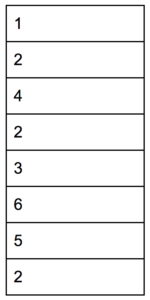In this example, you have a list of numbers ranging from 1-6. The unique values are the ones that appear only once in the list, without any duplications. The distinct values are all the different values in the list. The tables below show the unique and distinct values in this list.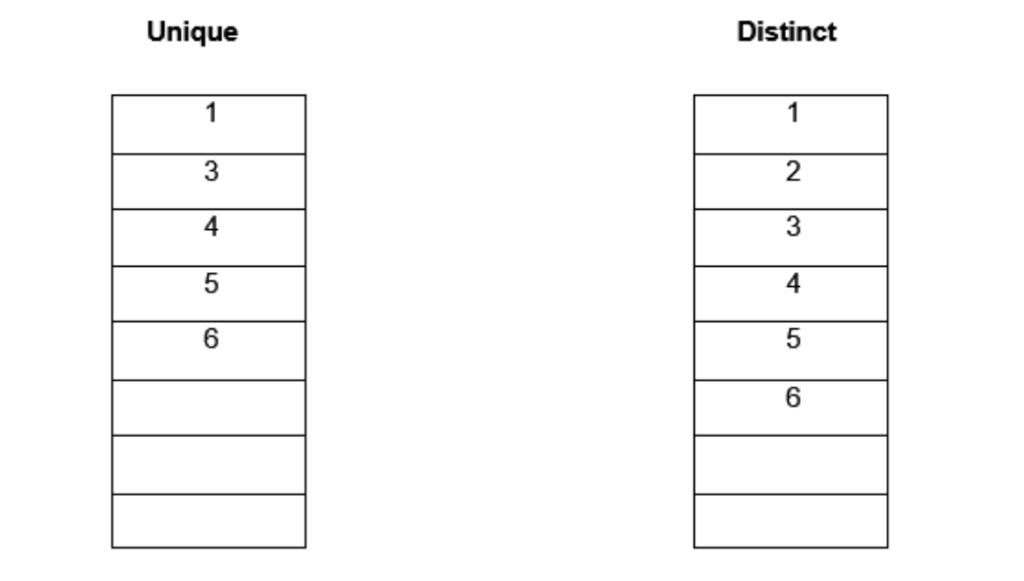### Count unique values in Excel

You can use the combination of the SUM and COUNTIF functions to count unique values in Excel. The syntax for this combined formula is = SUM(IF(1/COUNTIF(data, data)=1,1,0)). Here the COUNTIF formula counts the number of times each value in the range appears.

The resulting array looks like {1;2;1;1;1;1}. In the next step, you divide 1 by the resulting values. The IF function implements the logic such that if the values appear only once in the range, this step will generate 1, otherwise it will be a fraction value. The SUM function then sums all the values and returns the result. This is an array formula, so you have to assign it using Ctrl + Shift + Enter.

The following example contains a list of automobile products with their product ID and Names. You will count the unique items in this example.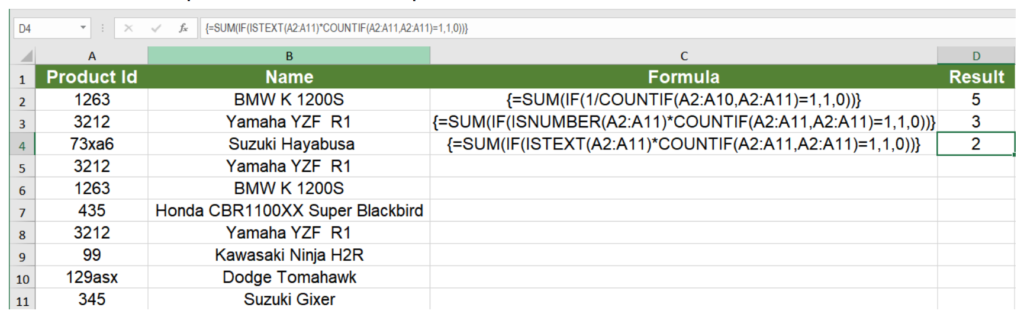`{=SUM(IF(1/COUNTIF(A2:A11,A2:A11)=1,1,0))}`

This counts the number of unique values in the list. It follows the syntax mentioned above and returns the count for unique items, which is 5.

`{=SUM(IF(ISNUMBER(A2:A11)*COUNTIF(A2:A11,A2:A11)=1,1,0))}`

This counts the number of unique numeric values in the list. The only difference with the previous formula is here is the nested ISNUMBER formula that makes sure that you only count the numeric values. Returns the number 3, which is the count of the unique numeric values.

`{=SUM(IF(ISTEXT(A2:A10)*COUNTIF(A2:A11,A2:A11)=1,1,0))}`

Works the same as the previous formula, counts the unique number of text values instead. The ISTEXT function is used to make sure that only the text values are counted. Returns the number 2.

### Count Distinct Values in Excel

Count Distinct Values using a Filter

You can extract the distinct values from a list using the Advanced Filter dialog box and use the ROWS function to count the unique values. To count the distinct values from the previous example:

• Select the range of cells A1:A11.
• Go to Data > Sort & Filter > Advanced.• In the Advanced Filter dialog box, click Copy to another location.
• Set both List Range and Criteria Range to \$A\$1:\$A\$11.
• Set Copy to to \$F\$2.
• Keep the Unique Records Only box checked. Click OK.• Column F will now contain the distinct values.
• Select H6.
• Enter the formula `=ROWS(F3:F9)`. Click Enter.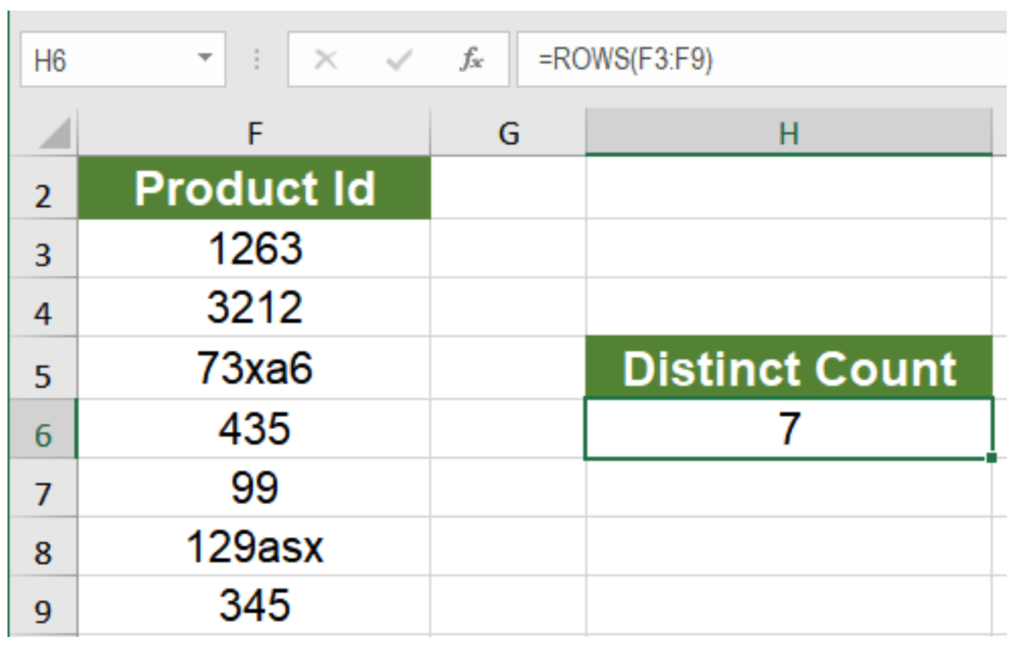This will show the count of distinct values, 7.

Count Distinct Values using Formulas

You can use the combination of the IF, MATCH, LEN and FREQUENCY functions with the SUM function to count distinct values.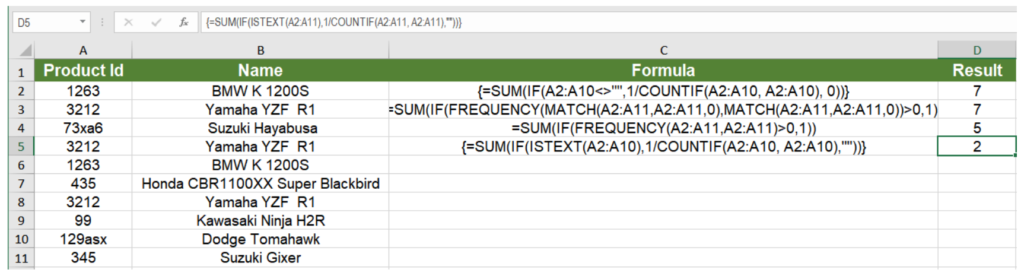`{=SUM(IF(A2:A11<>"",1/COUNTIF(A2:A11, A2:A11), 0))}`

Counts the number of distinct values between cells A2 to A11. Like the unique count, here the COUNTIF function returns a count for each individual value which is then used as a denominator to divide 1. The returning values are then summed if they are not 0. This gives you a count for the distinct values, regardless of their types.

`=SUM(IF(FREQUENCY(MATCH(A2:A11,A2:A11,0),MATCH(A2:A11,A2:A11,0))>0,1))`

Does the same thing as the previous formula. The only differences being the use of the FREQUENCY and MATCH functions. The frequency function returns the number of occurrences for a value for the first occurrence. For the next occurrence of that value, it returns 0. The MATCH function is used to return the position of a text value in the range. These are returned as then used as an argument to the FREQUENCY function which gives us a count of the total number of distinct values.

`=SUM(IF(FREQUENCY(A2:A11,A2:A11)>0,1))`

Counts the number of distinct numeric values. As the FREQUENCY function ignores text and blanks, it returns 5, which is the number of numeric values.

`{=SUM(IF(ISTEXT(A2:A11),1/COUNTIF(A2:A11, A2:A11),""))}`

Returns the count of distinct text values in a range. Like the first example, this counts the distinct values, but the ISTEXT function makes sure only the text values are taken into count.

Count Distinct Values using a Pivot Table

You can also count distinct values in Excel using a pivot table. To find the distinct count of the bike names from the previous example:

To count the distinct items using a pivot table:

• Select cells A1:B11. Go to Insert > Pivot Table.
• In the dialog box that pops up, check New Worksheet and Add this to the Data Model.• Drag the Product ID field to Rows, Names field to Values in the PivotTable Fields.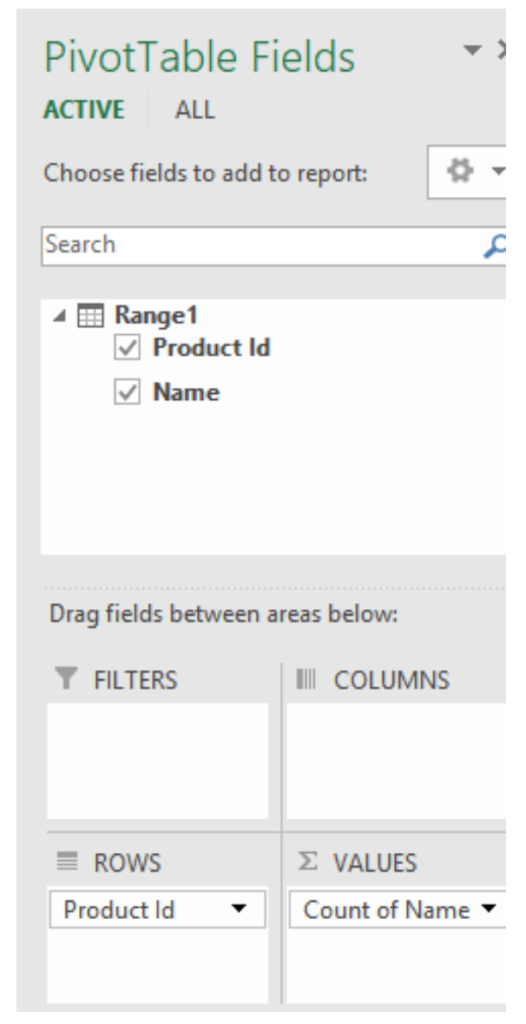• Right-click on any value in column B. Go to Value Field Settings. In the Summarize Values By tab, go to Summarize Value field by and set it to Distinct Count.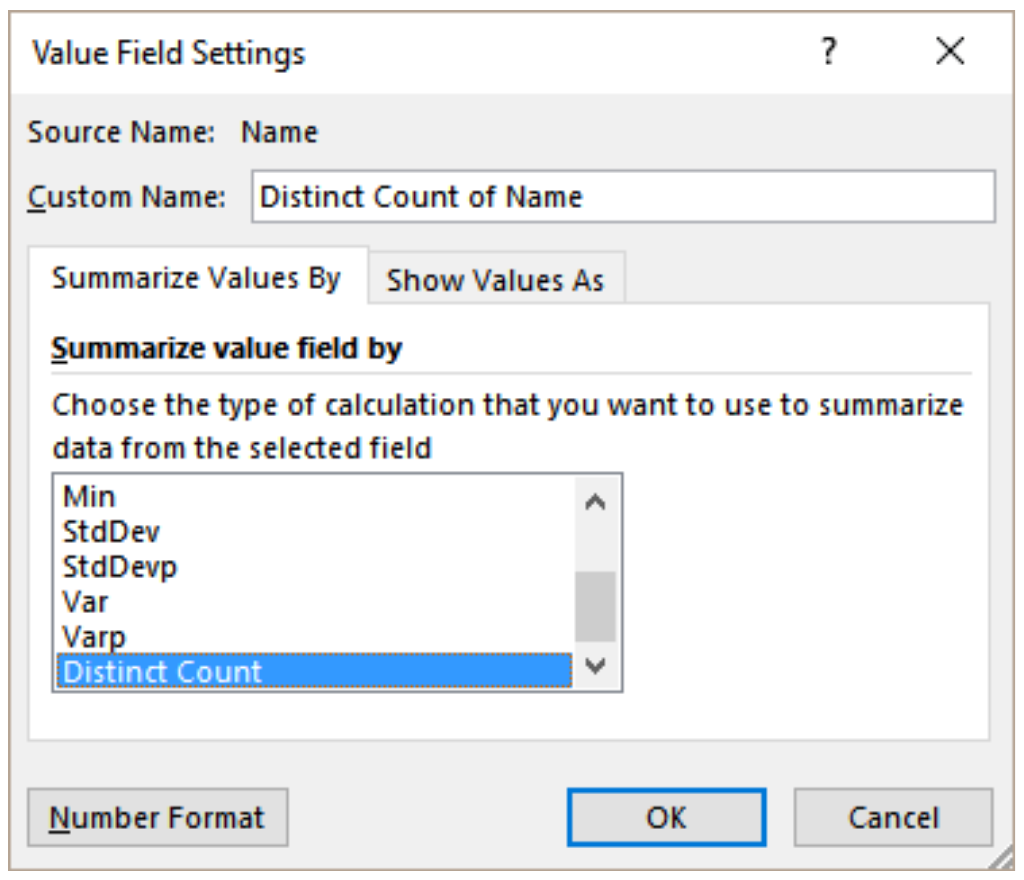This will show the distinct count 7 in cell B11.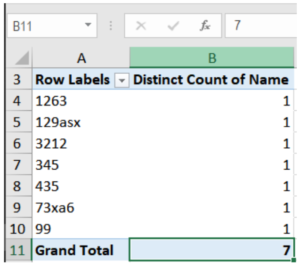Still need some help with Excel formatting or have other questions about Excel? Connect with a live Excel expert here for some 1 on 1 help. Your first session is always free.

### Did this post not answer your question? Get a solution from connecting with the expert.Another blog reader asked this question today on Excelchat:
Solution examplesI have a file in which I need to somehow use a conditional formatting for the same names of the data available, or if there is any other way to calculate the frequency of one particular name appearing.
Solved by K. F. in 51 minsHello, I am trying to create a total color column? Can anyone help?
Solved by I. A. in 60 minsI need to convert the sum of 3 cells into a single number, ie if cell a and b = 200, 150 and cell c = 0, i only want cells with a value greater than zero to be counted until such a time that cell gets a value
Solved by V. L. in 20 minsHi, I am trying to get a sum of three separate cells in excel by typing "=H45+H23+H37"...and eventhough only one of the cells shows "0.00", and the remaining two cells have positive balance, the result I get is "0.00".................
Solved by K. L. in 18 minsHi , I have two columns in XLS and it has value . I need to check if either of the two value is true along with third column which has value . I need to count such columns
Solved by Z. D. in 40 mins## Subscribe to Excelchat.coAnother blog reader asked this question today on Excelchat: